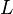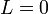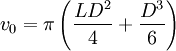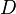# Difference between revisions of "Hard spherocylinders"

The hard spherocylinder model consists of an impenetrable cylinder, capped at both ends by hemispheres whose diameters are the same as the diameter of the cylinder. The hard spherocylinder model has been studied extensively because of its propensity to form both nematic and smectic liquid crystalline phases  as well as forming a plastic crystal phase for short$L$  . One of the first studies of hard spherocylinders was undertaken by Cotter and Martire  using scaled-particle theory, and one of the first simulations was in the classic work of Jacques Vieillard-Baron . In the limit of zero diameter the hard spherocylinder becomes a line segment, often known as the hard rod model, and in the limit$L=0$ one has the hard sphere model. A closely related model is that of the oblate hard spherocylinder.

## Volume

The molecular volume of the spherocylinder is given by$v_0 = \pi \left( \frac{LD^2}{4} + \frac{D^3}{6} \right)$

where$L$ is the length of the cylindrical part of the spherocylinder and$D$ is the diameter.

## Minimum distance

The minimum distance between two spherocylinders can be calculated using an algorithm published by Vega and Lago . The source code can be found here. Such an algorithm is essential in, for example, a Monte Carlo simulation, in order to check for overlaps between two sites.

The original code did not give the symmetry property of the distance for almost parallel rods. The revised algorithm in C for systems with periodic boundary conditions can be found here.

## Virial coefficients

Virial coefficients of the hard spherocylinder model   .

## Phase diagram

Phase diagram of the hard spherocylinder model